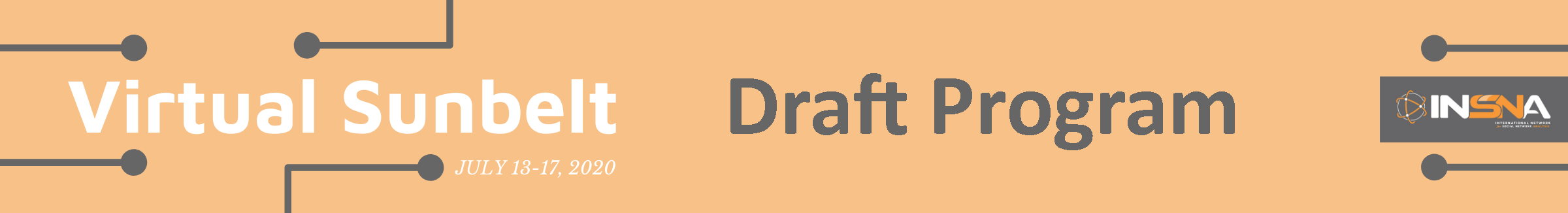## Utilizing Blockmodeling, Clustering, and Heatmaps to Visualize Multinomial Logistic Regression Models

### Kelsey Gonzalez

#### Contact: kelseygonzalez@email.arizona.edu

Multinomial logistic regression models are notoriously difficult to substantively interpret due to the multiple layers of reference categories. This paper addresses this difficulty often found in social science research by creating a visualization technique for multinomial logit models inspired by blockmodeling using the R programming language. Using the base multinomial logistic regression model results, this method mines the predicted probabilities of each variable level. I employ a method of quasi-blockmodeling through agglomerative hierarchical clustering to sort predictors by similarity of effects on the dependent variable. The sorted predicted probabilities are visualized using heatmaps which display the deviation from the mean for each normalized column. The resulting methodological technique aims to help social science researchers extrapolate general trends and uncover relationships within the context of multinomial logistic regression instead of becoming too enmeshed in the multi-layered reference categories and p-value significance.

← Schedule